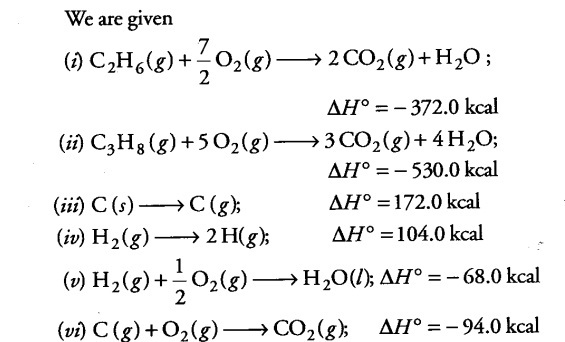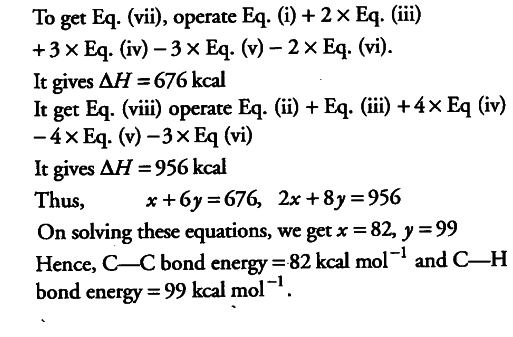# Sing the data (all values are in kilocalories per mole at 25° C)

Using the data (all values are in kilocalories per mole at 25° C) given below, calculate the bond energy of C—C and C—H bonds.
∆H° combustion (ethane) = -372.0
∆H° combustion(propane) = -530.0
∆H°for C (graphite) -----> C(g) = 172.0
∆fH° of \$H { 2 }O\$ (l) = -68.0
∆H° of \${{CO}
{2}}\$ (g) = -94.0